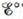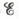# The overall reaction in the lead storage battery is Pb ( s ) + PbO 2 ( s ) + 2 H + ( a q ) + 2 HSO 4 − ( a q ) → 2 PbSO 4 ( s ) + 2 H 2 O ( l ) a. For the cell reaction ∆ H ° = −315.9 kJ and ∆ S ° = 263.5 J/K. Calculate at −20.°C. Assume ∆ H ° and ∆ S ° do not depend on temperature. b. Calculate at – 20.°C when [HSO 4 − ] = [H + ] = 4.5 M . c. Consider your answer to Exercise 71. Why does it seem that batteries fail more often on cold days than on warm days?### Chemistry: An Atoms First Approach

2nd Edition
Steven S. Zumdahl + 1 other
Publisher: Cengage Learning
ISBN: 9781305079243

#### Solutions

Chapter
Section### Chemistry: An Atoms First Approach

2nd Edition
Steven S. Zumdahl + 1 other
Publisher: Cengage Learning
ISBN: 9781305079243
Chapter 17, Problem 142CP
Textbook Problem
10 views

## The overall reaction in the lead storage battery is Pb ( s )   +   PbO 2 ( s )   +   2 H + ( a q )   +   2 HSO 4 − ( a q )   →   2 PbSO 4 ( s ) +   2 H 2 O ( l ) a. For the cell reaction ∆H° = −315.9 kJ and ∆S° = 263.5 J/K. Calculateat −20.°C. Assume ∆H° and ∆S° do not depend on temperature.b. Calculateat – 20.°C when [HSO4−] = [H+] = 4.5 M.c. Consider your answer to Exercise 71. Why does it seem that batteries fail more often on cold days than on warm days?

(a)

Interpretation Introduction

Interpretation:

The overall reaction taking place in lead storage battery is given. various questions based on the given battery have to be answered.

Concept introduction:

The device that contains one or more electrochemical cells that is used to provide electrical energy to the different electrical devices is called a battery.

The relationship between reduction potential and standard reduction potential value and activities of species present in an electrochemical cell at a given temperature is given by the Nernst equation.

The value of Ecell is calculated using Nernst formula,

E=E°(RTnF)ln(Q)

At room temperature, the above equation specifies as,

E=E°(0.0591n)log(Q)

The relationship between Gibbs free energy change and cell potential is given by the formula,

ΔG°=nFE°cell

The relation between ΔG° , ΔH° and ΔS° is given as,

ΔG°=ΔH°TΔS°

To determine: The value of E° at the given reaction conditions.

The value of E° is 1.98V_ .

### Explanation of Solution

Given

The value of ΔH° is 315.9kJ .

The value of ΔS° is 263.5J/K .

The given temperature is 20°C .

The conversion of kJ to J is done as,

1kJ=1000J

Conversion of 315.9kJ to J is done as,

315.9kJ=315.9×1000J=-315.9×103J

The conversion of degree Celsius (°C) into Kelvin (K) is done as,

T(K)=T(°C)+273.15

Hence, the conversion of 20°C to K is done as,

T(K)=20°C+273.15=253.15K

The reaction taking place at the cathode is,

PbO2+HSO4+3H++2ePbSO4+2H2O

The reaction taking place at the anode is,

Pb+HSO4PbSO4+H++2e

The reaction taking place in lead-storage battery is,

Pb(s)+PbO2(s)+2H+(aq)+2HSO4(aq)2PbSO4(s)+2H2O(l)

The relation between ΔG° , ΔH° and ΔS° is given as,

ΔG°=ΔH°TΔS° (1)

Where,

• ΔG° is the standard Gibbs free energy change

(b)

Interpretation Introduction

Interpretation:

The overall reaction taking place in lead storage battery is given. various questions based on the given battery have to be answered.

Concept introduction:

The device that contains one or more electrochemical cells that is used to provide electrical energy to the different electrical devices is called a battery.

The relationship between reduction potential and standard reduction potential value and activities of species present in an electrochemical cell at a given temperature is given by the Nernst equation.

The value of Ecell is calculated using Nernst formula,

E=E°(RTnF)ln(Q)

At room temperature, the above equation specifies as,

E=E°(0.0591n)log(Q)

The relationship between Gibbs free energy change and cell potential is given by the formula,

ΔG°=nFE°cell

The relation between ΔG° , ΔH° and ΔS° is given as,

ΔG°=ΔH°TΔS°

To determine: The value of E at the given concentration of ions.

The value of E is 2.05V_ .

(c)

Interpretation Introduction

Interpretation:

The overall reaction taking place in lead storage battery is given. various questions based on the given battery have to be answered.

Concept introduction:

The device that contains one or more electrochemical cells that is used to provide electrical energy to the different electrical devices is called a battery.

The relationship between reduction potential and standard reduction potential value and activities of species present in an electrochemical cell at a given temperature is given by the Nernst equation.

The value of Ecell is calculated using Nernst formula,

E=E°(RTnF)ln(Q)

At room temperature, the above equation specifies as,

E=E°(0.0591n)log(Q)

To determine: The reason of failure of batteries in cold days than on warm days.

The decrease in value of cell potential with decrease in temperature leads to the failure of batteries in cold days than on warm days.

### Still sussing out bartleby?

Check out a sample textbook solution.

See a sample solution

#### The Solution to Your Study Problems

Bartleby provides explanations to thousands of textbook problems written by our experts, many with advanced degrees!

Get Started

Find more solutions based on key concepts
Characterize gases, liquids, and solids in terms of compressibility and fluidity.

General Chemistry - Standalone book (MindTap Course List)

Today, an estimated 69 percent of the adults in the United States are overweight or obese.

Nutrition: Concepts and Controversies - Standalone book (MindTap Course List)

22-19 Why are all amino acids solids at room temperature?

Introduction to General, Organic and Biochemistry

A car initially traveling eastward turns north by traveling in a circular path at uniform speed as shown in Fig...

Physics for Scientists and Engineers, Technology Update (No access codes included)

True or false? The plasma membrane is the outermost component of all cells. Explain.

Biology: The Unity and Diversity of Life (MindTap Course List)

Intensities being equal, which color of light moves farthest through seawater. Least far?

Oceanography: An Invitation To Marine Science, Loose-leaf Versin